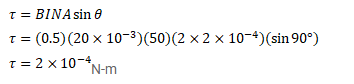# A moving-coil galvanometer has a 50-turn coil of size 2cm×2cm.

Question:

A moving-coil galvanometer has a 50 -turn coil of size $2 \mathrm{~cm} \times 2 \mathrm{~cm}$. It is suspended between the magnetic poles producing a magnetic field of $0.5 \mathrm{~T}$. Find the torque on the coil due to the magnetic field when a current of $20 \mathrm{~mA}$ passes through it.

Solution: